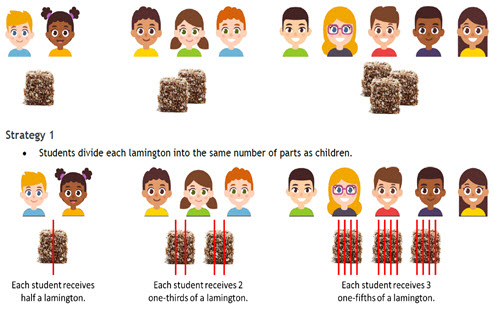Go to website

# reSolve: Lamingtons

This sequence of three lessons explores the mathematical idea that fractions represent division. In the first lesson, students are invited to solve a problem involving fair sharing of different plates of lamingtons. Students explore how the denominator represents how many shares and the numerator represents the number being shared. In subsequent lessons, students use unit fractions to compare quantities and further extend the sharing of different numbers of lamingtons across different-sized groups. Each lesson is outlined in detail including curriculum links, vocabulary, materials needed, sample answers, discussion points and student resources. This sequence is part of the reSolve: Mathematics by Inquiry program.

Year level(s) Year 5
Audience Teacher
Purpose Teaching resource
Teaching strategies and pedagogical approaches Mathematics investigation
Keywords proportional reasoning, unit fractions, denominators, fractions

## Curriculum alignment

Curriculum connections Numeracy
Strand and focus Number, Build understanding, Apply understanding
Topics Fractions
AC: Mathematics content descriptions
ACMNA102 Compare and order common unit fractions and locate and represent them on a number line
ACMNA103 Investigate strategies to solve problems involving addition and subtraction of fractions with the same denominator
National numeracy learning progression Interpreting fractions - InF5, InF6, InF7